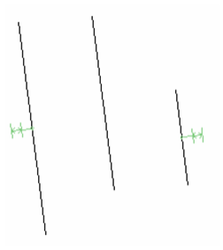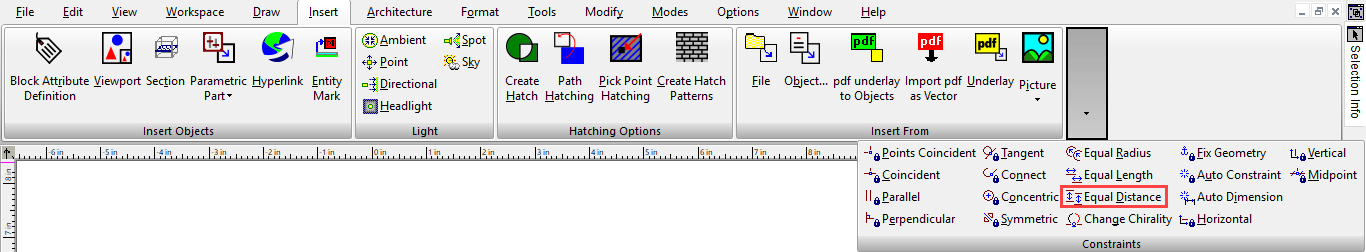Sets the distance between two lines to the same between two other lines. Sets of lines are also made parallel.

1. Start with four lines. Activate the Equal Distance constraint and select the first line in the first set.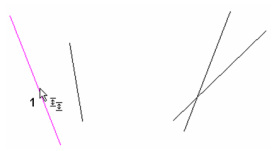1. Select the second line in the first set. This establishes the distance for the next set.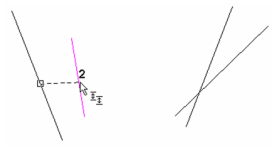1. Select the first line in the second set.1. Select the second line in the second set.The first set and second set of lines are made parallel. The distance between the lines in the second set matches the distance in the first set.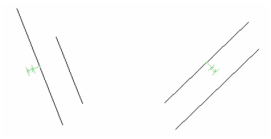1. If you edit any line, the equal length constraint will be maintained.1. You can also apply this constraint to a row of lines or points. Select the first and second lines.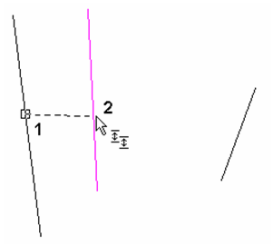1. The third line is the same as the second, then select the fourth.The result is three lines, parallel and separated by the same distance.